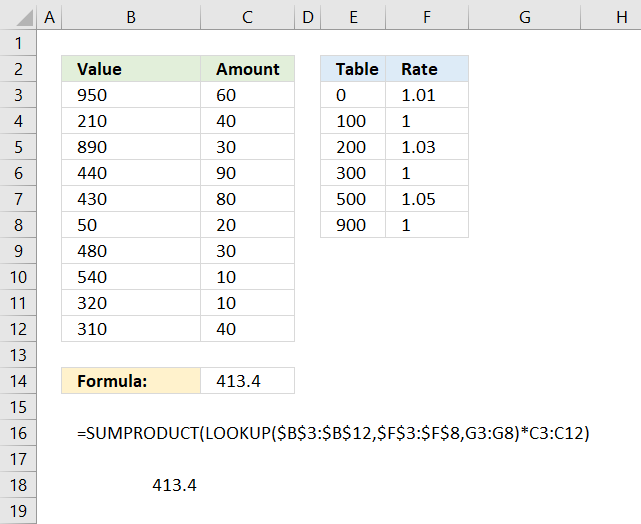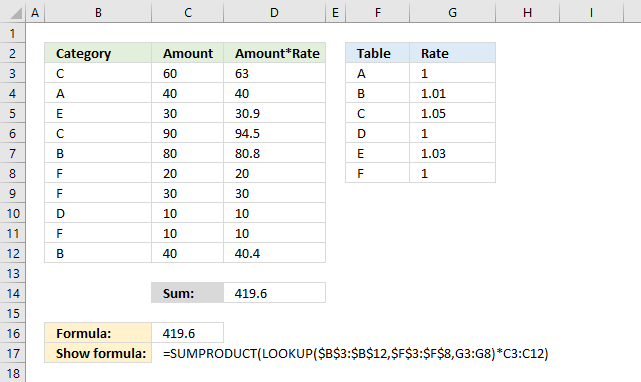Author: Oscar Cronquist Article last updated on December 12, 2017I have demonstrated in a previous post how to simplify nested IF functions, in this article I will show you how to simplify your SUMPRODUCT formulas regarding multiple criteria.

In most cases, there is no need for IF functions in SUMPRODUCT formulas, this is true in this case as well, the criteria below are complicated to build with IF functions.

0 <= value < 100 Rate: 1.01
100 <= value < 200 Rate: 1
200 <= value < 300 Rate: 1.03
300 <= value < 500 Rate: 1
500 <= value < 900 Rate: 1.05
900 <= value Rate: 1

If a value in column B is matching one of the above ranges the corresponding rate is used.

However, it can be easily simplified using the LOOKUP function. The following formula is entered in cell C14 in the image above.

=SUMPRODUCT(LOOKUP(B3:B12,E3:E8,F3:F8)*C3:C12)

A small table is easy to build, shown in column E and F. The LOOKUP function requires the values in E3:E8 to be sorted in an ascending order for it to work properly.

Instead of using one lookup value in the first argument I am using an entire cell range.

LOOKUP(B3:B12,E3:E8,F3:F8)

The rate is determined by the value in B3:B12.

LOOKUP({950; 210; 890; 440; 430; 50; 480; 540; 320; 310},{0; 100; 200; 300; 500; 900},{1.01; 1; 1.03; 1; 1.05; 1})

The LOOKUP function matches the values in B3:B12 to the values in F3:F8 and returns the corresponding value from G3:G8 simultaneously.

{1; 1.03; 1.05; 1; 1; 1.01; 1; 1.05; 1; 1}

Now we know which rates to use, it is now possible to multiply the amounts.

{1; 1.03; 1.05; 1; 1; 1.01; 1; 1.05; 1; 1}*C3:C12

becomes

{1; 1.03; 1.05; 1; 1; 1.01; 1; 1.05; 1; 1}*{60; 40; 30; 90; 80; 20; 30; 10; 10; 40}

and returns

{60;41.2;31.5;90;80;20.2;30;10.5;10;40}

Lastly, the SUMPRODUCT function adds all numbers and returns a total.

SUMPRODUCT({60;41.2;31.5;90;80;20.2;30;10.5;10;40})

and returns 413.4 in cell C14.

So what would the equivalent formula look like using IF functions?

=SUMPRODUCT(IF((B3:B12>=0)*(B3:B12<100), 1.01, IF((B3:B12>=200)*(B3:B12<300), 1.03, IF((B3:B12>=500)*(B3:B12<900), 1.05, 1)))*C3:C12)You can use letters also with the LOOKUP function, remember to have them sorted from A to Z, the image above shows you this in F3:F8.

Column D is a column to verify the calculation, you don't need it.

### Get excel *.xlsx file

SUMPRODUCT - nested IF functions.xlsx